GeeksforGeeks App
Open AppBrowser
Continue

# Split dataframe in Pandas based on values in multiple columns

In this article, we are going to see how to divide a dataframe by various methods and based on various parameters using Python. To divide a dataframe into two or more separate dataframes based on the values present in the column we first create a data frame.

## Python3

 `# importing pandas as pd``import` `pandas as pd`` ` ` ` `# dictionary of lists``dict` `=` `{``'First_Name'``: [``"Aparna"``, ``"Pankaj"``, ``"Sudhir"``, ``                       ``"Geeku"``, ``"Anuj"``, ``"Aman"``,``                       ``"Madhav"``, ``"Raj"``, ``"Shruti"``],``        ``'Last_Name'``: [``"Pandey"``, ``"Gupta"``, ``"Mishra"``, ``                      ``"Chopra"``, ``"Mishra"``, ``"Verma"``, ``                      ``"Sen"``, ``"Roy"``, ``"Agarwal"``],``        ``'Email_ID'``: [``"apandey@gmail.com"``, ``"pankaj@gmail.com"``,``                     ``"sumishra23@gmail.com"``, ``"cgeeku@yahoo.com"``,``                     ``"anuj24@gmail.com"``, ``"amanver@yahoo.com"``,``                     ``"madhav1998@gmail.com"``, ``"rroy7@gmail.com"``,``                     ``"sagarwal36@gmail.com"``],``        ``'Degree'``: [``"MBA"``, ``"BCA"``, ``"M.Tech"``, ``"MBA"``, ``"B.Sc"``,``                   ``"B.Tech"``, ``"B.Tech"``, ``"MBA"``, ``"M.Tech"``],``        ``'Score'``: [``90``, ``40``, ``75``, ``98``, ``94``, ``90``, ``80``, ``90``, ``95``]}`` ` `# creating dataframe``df ``=` `pd.DataFrame(``dict``)`` ` `print``(df)`

Output: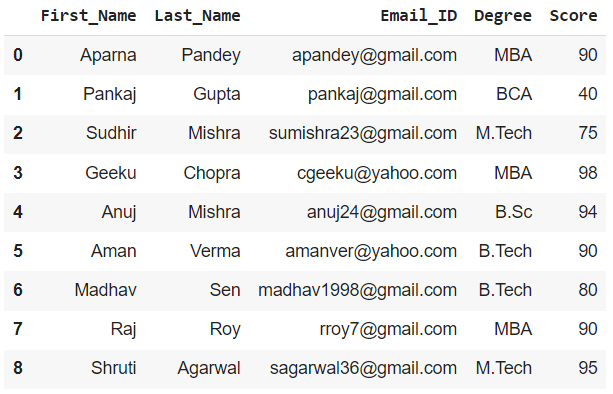## Method 1: By Boolean Indexing

We can create multiple dataframes from a given dataframe based on a certain column value by using the boolean indexing method and by mentioning the required criteria.

Example 1: Creating a dataframe for the students with Score >= 80

## Python3

 `# creating a new dataframe by applying the required ``# conditions in [] ``df1 ``=` `df[df[``'Score'``] >``=` `80``]`` ` `print``(df1)`

Output: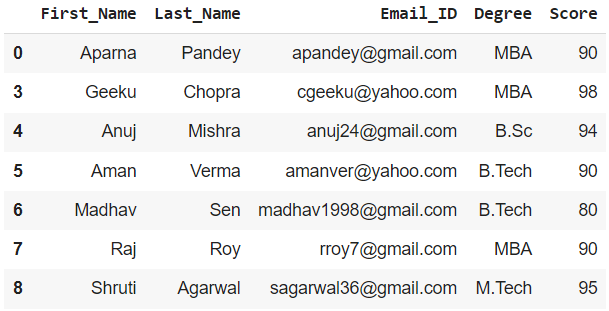Example 2: Creating a dataframe for the students with Last_Name as Mishra

## Python3

 `# Creating on the basis of Last_Name``dfname ``=` `df[df[``'Last_Name'``] ``=``=` `'Mishra'``]`` ` `print``(dfname)`

Output: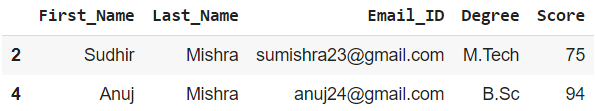We can do the same for other columns as well by putting the appropriate condition

## Method 2: Boolean Indexing with mask variable

We create a mask variable for the condition of the column in the previous method

Example 1: To get dataframe of students with Degree as MBA

## Python3

 `# creating the mask variable with appropriate``# condition``mask_var ``=` `df[``'Degree'``] ``=``=``'MBA'`` ` `# creating a dataframe``df1_mask ``=` `df[mask_var]`` ` `print``(df1_mask)`

Output :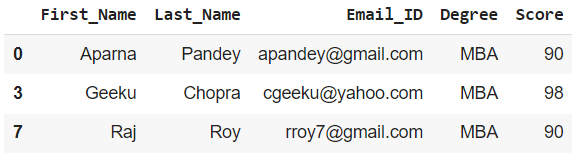Example 2: To get a dataframe for the rest of the students

To get the rest of the values in a dataframe we can simply invert the mask variable by adding a ~(tilde) after it.

## Python3

 `# creating dataframe with inverted mask variable``df2_mask ``=` `df[~mask_var]`` ` `print``(df2_mask)`

Output :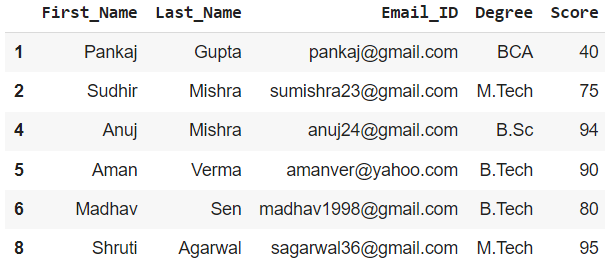## Method 3: Using groupby() function

Using groupby() we can group the rows using a specific column value and then display it as a separate dataframe.

Example 1: Group all Students according to their Degree and display as required

## Python3

 `# Creating an object using groupby``grouped ``=` `df.groupby(``'Degree'``)`` ` `# the return type of the object 'grouped' is ``# pandas.core.groupby.generic.DataFrameGroupBy.`` ` `# Creating a dataframe from the object using get_group().``# dataframe of students with Degree as MBA.``df_grouped ``=` `grouped.get_group(``'MBA'``)`` ` `print``(df_grouped)`

Output: dataframe of students with Degree as MBA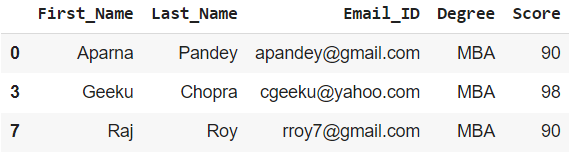Example 2: Group all Students according to their Score and display as required

## Python3

 `# Creating another object using groupby``grouped2 ``=` `df.groupby(``'Score'``)`` ` `# the return type of the object 'grouped2' is ``# pandas.core.groupby.generic.DataFrameGroupBy.`` ` `# Creating a dataframe from the object ``# using get_group() dataframe of students``# with Score = 90``df_grouped2 ``=` `grouped2.get_group(``90``)`` ` `print``(df_grouped2)`

Output: dataframe of students with Score = 90.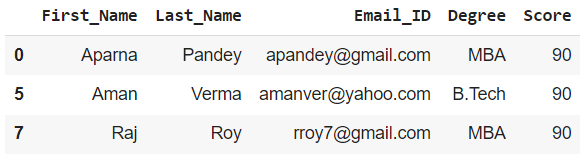My Personal Notes arrow_drop_up